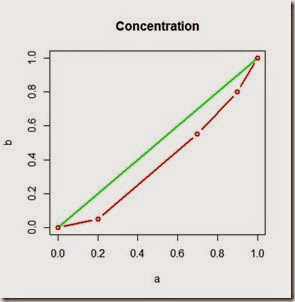Want to share your content on R-bloggers? click here if you have a blog, or here if you don't.

Another subject we took in the statistics class was the Gini index.

Gini index or ratio or coefficient is used to calculate how much a certain transferable phenomenon such as income or stocks for instance, is concentrated.

For example, say you are evaluating a company and you’d like to know more about how the shares are divided among the shareholders. You could use Gini index for that!

I’ve calculated the index using R and random data you can download here. In case you’d like to know more about Gini index check here.Here my simple R implementation of the index.

Here below are the resultsIt looks like the data I used shows a 24% concentration. Cool!